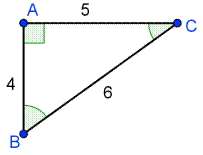# Given the triangle below, which of the following is a correct statement?

Given the triangle below, which of the following is a correct statement?
A. csc right triangle B right triangle equals fraction 3 over 2
B. csc right triangle C right triangle equals fraction 3 over 2
C. csc right triangle B right triangle equals fraction 6 over 5
D. csc right triangle C right triangle equals fraction 5 over 6